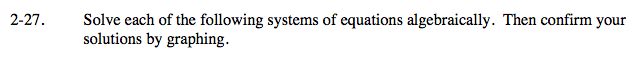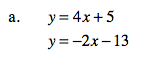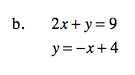### Home > CAAC > Chapter 14 > Lesson 14.2.1.2 > Problem2-27

2-27.
1. Solve each of the following systems of equations algebraically. Then confirm your solutions by graphing. Homework Help ✎

1. y = 4x + 5
y = −2x − 13

2. 2x + y = 9
y = −x + 4Substitute 4x + 5 for y.

4x + 5 = −2x − 13See part (a).

Solve for x.

x = −3

Substitute −3 for x.

y = −12 + 5

Solve for y.

y = −7

(−3, −7)